# 创建一个不会赔钱的比特币交易机器人

Author: , Created: 2019-06-27 10:58:40, Updated: 2019-07-08 15:58:28

## 让我们使用人工智能中的强化学习来制作一个数字货币交易机器人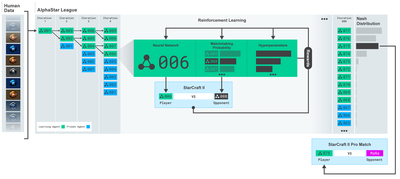## 计划1.为我们的机器人创建gym环境以供其进行机器学习

2.渲染一个简单而优雅的可视化环境

3.训练我们的机器人，使其学习一个可获利的交易策略

## 入门

``````import gym
import pandas as pd
import numpy as np
from gym import spaces
from sklearn import preprocessing
``````

``````class BitcoinTradingEnv(gym.Env):
"""A Bitcoin trading environment for OpenAI gym"""
metadata = {'render.modes': ['live', 'file', 'none']}
scaler = preprocessing.MinMaxScaler()
viewer = None
def __init__(self, df, lookback_window_size=50,
commission=0.00075,
initial_balance=10000
serial=False):
self.df = df.dropna().reset_index()
self.lookback_window_size = lookback_window_size
self.initial_balance = initial_balance
self.commission = commission
self.serial = serial
# Actions of the format Buy 1/10, Sell 3/10, Hold, etc.
self.action_space = spaces.MultiDiscrete([3, 10])
# Observes the OHCLV values, net worth, and trade history
self.observation_space = spaces.Box(low=0, high=1, shape=(10, lookback_window_size + 1), dtype=np.float16)
``````

``````def reset(self):
self.balance = self.initial_balance
self.net_worth = self.initial_balance
self.btc_held = 0
self._reset_session()
self.account_history = np.repeat([
[self.net_worth],
,
,
,

], self.lookback_window_size + 1, axis=1)
return self._next_observation()
``````

## 交易会话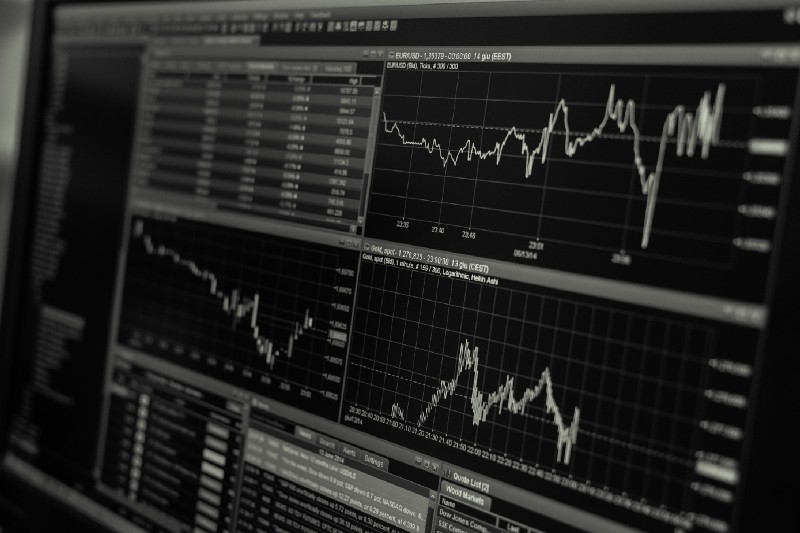``````MAX_TRADING_SESSION = 100000 ＃ ~2个月

``````

``````def _reset_session(self):
self.current_step = 0
if self.serial:
self.steps_left = len(self.df) - self.lookback_window_size - 1
self.frame_start = self.lookback_window_size
else:
self.frame_start = np.random.randint(self.lookback_window_size, len(self.df) - self.steps_left)
self.active_df = self.df[self.frame_start - self.lookback_window_size:self.frame_start + self.steps_left]
``````

## 通过机器人的眼睛观察到的OpenCV可视化环境的观察

• 重要的是只扩展机器人到目前为止所观察到的数据，以防止超前偏差。
``````def _next_observation(self):
end = self.current_step + self.lookback_window_size + 1
obs = np.array([
self.active_df['Open'].values[self.current_step:end],
self.active_df['High'].values[self.current_step:end],
self.active_df['Low'].values[self.current_step:end],
self.active_df['Close'].values[self.current_step:end],
self.active_df['Volume_(BTC)'].values[self.current_step:end],])
scaled_history = self.scaler.fit_transform(self.account_history)
obs = np.append(obs, scaled_history[:, -(self.lookback_window_size + 1):], axis=0)
return obs
``````

### 采取行动

``````def step(self, action):
current_price = self._get_current_price() + 0.01
self._take_action(action, current_price)
self.steps_left -= 1
self.current_step += 1
if self.steps_left == 0:
self.balance += self.btc_held * current_price
self.btc_held = 0
self._reset_session()
obs = self._next_observation()
reward = self.net_worth
done = self.net_worth <= 0
return obs, reward, done, {}
``````

``````def _take_action(self, action, current_price):
action_type = action
amount = action / 10
btc_bought = 0
btc_sold = 0
cost = 0
sales = 0
if action_type < 1:
btc_bought = self.balance / current_price * amount
cost = btc_bought * current_price * (1 + self.commission)
self.btc_held += btc_bought
self.balance -= cost
elif action_type < 2:
btc_sold = self.btc_held * amount
sales = btc_sold * current_price  * (1 - self.commission)
self.btc_held -= btc_sold
self.balance += sales
``````

``````if btc_sold > 0 or btc_bought > 0:
'step': self.frame_start+self.current_step,
'amount': btc_sold if btc_sold > 0 else btc_bought,
'total': sales if btc_sold > 0 else cost,
'type': "sell" if btc_sold > 0 else "buy"
})
self.net_worth = self.balance + self.btc_held * current_price
self.account_history = np.append(self.account_history, [
[self.net_worth],
[btc_bought],
[cost],
[btc_sold],
[sales]
], axis=1)
``````

## 观看我们的机器人交易

``````from datetime import datetime
``````

``````date_labels = np.array([datetime.utcfromtimestamp(x).strftime('%Y-%m-%d %H:%M') for x in self.df['Timestamp'].values[step_range]])
``````

``````def render(self, mode='human', **kwargs):
if mode == 'human':
if self.viewer == None:
kwargs.get('title', None))
self.viewer.render(self.frame_start + self.current_step,
self.net_worth,
window_size=self.lookback_window_size)
``````## 训练时间

``````slice_point = int(len(df) - 100000)
train_df = df[:slice_point]
test_df = df[slice_point:]
``````

``````train_env = DummyVecEnv([lambda: BitcoinTradingEnv(train_df, commission=0, serial=False)])
test_env = DummyVecEnv([lambda: BitcoinTradingEnv(test_df, commission=0, serial=True)])
``````

``````model = PPO2(MlpPolicy,
train_env,
verbose=1,
tensorboard_log="./tensorboard/")
model.learn(total_timesteps=50000)
``````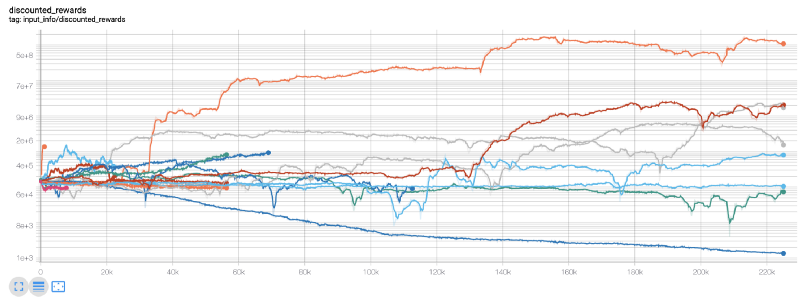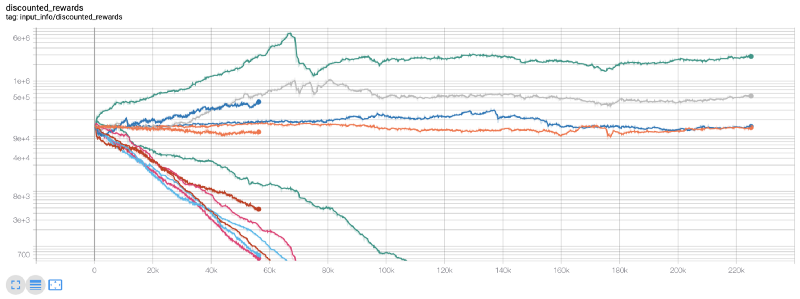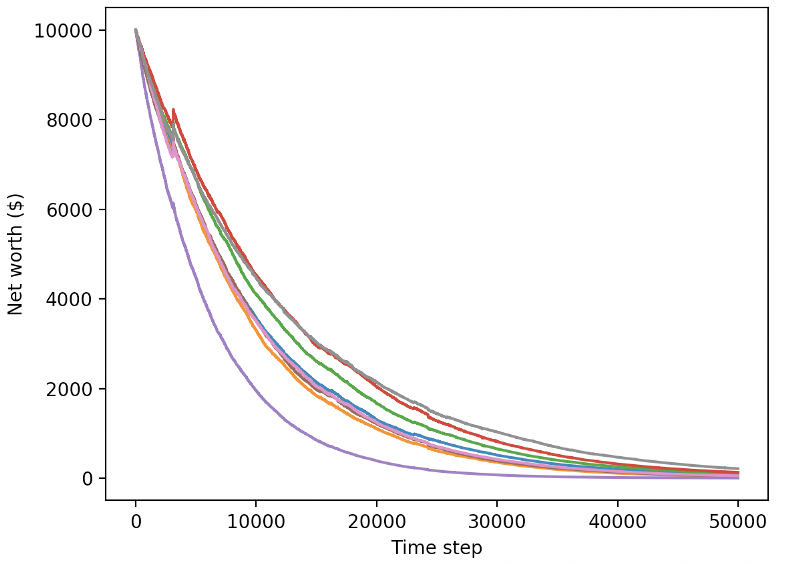``````reward = self.net_worth - prev_net_worth
``````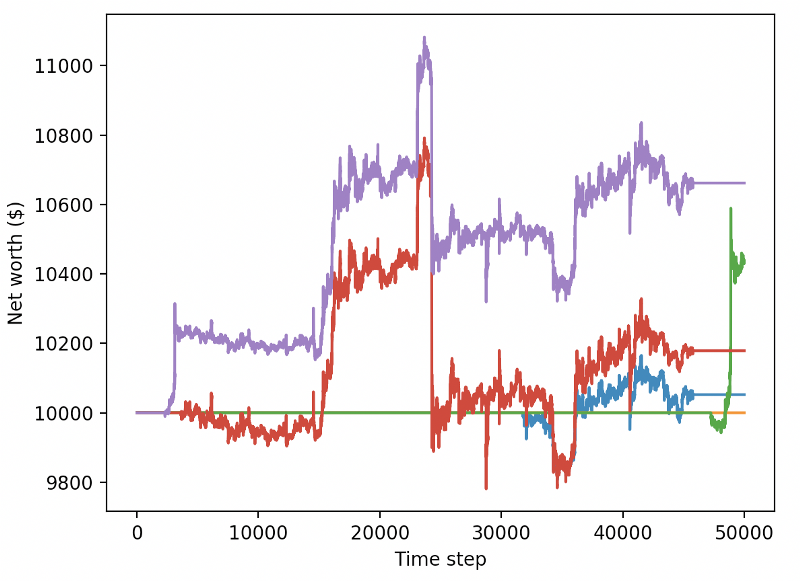## 结论

1.使用OpenAI的gym从零开始创建比特币交易环境。

2.使用Matplotlib构建该环境的可视化。

3.使用简单的交叉验证对我们的机器人进行训练和测试。

4.略微调整我们的机器人以实现盈利

More